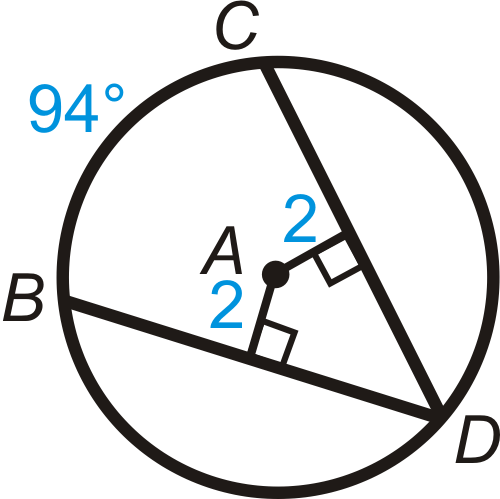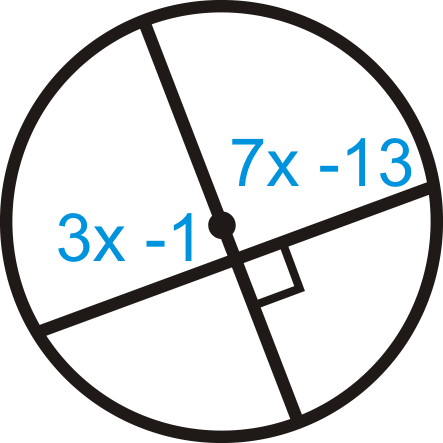# 6.12: Chords and Central Angle Arcs

•• Contributed by CK12
• CK12

Arcs determined by angles whose vertex is the center of a circle and chords (segments that connect two points on a circle).

## Chords in Circles

### Chord Theorems

1. Chord Theorem #1: In the same circle or congruent circles, minor arcs are congruent if and only if their corresponding chords are congruent.Figure $$\PageIndex{1}$$

In both of these pictures, $$\overline{BE}\cong \overline{CD}$$ and $$\widehat{BE}\cong \widehat{CD}$$.

2. Chord Theorem #2: The perpendicular bisector of a chord is also a diameter.Figure $$\PageIndex{2}$$

If $$\overline{AD}\perp \overline{BC}$$ and $$\overline{BD}\cong \overline{DC}$$ then $$\overline{EF}$$ is a diameter.

3. Chord Theorem #3: If a diameter is perpendicular to a chord, then the diameter bisects the chord and its corresponding arc.Figure $$\PageIndex{3}$$

If $$\overline{EF}\perp \overline{BC}$$, then $$\overline{BD}\cong \overline{DC}$$

4. Chord Theorem #4: In the same circle or congruent circles, two chords are congruent if and only if they are equidistant from the center.Figure $$\PageIndex{4}$$

The shortest distance from any point to a line is the perpendicular line between them. If $$FE=EG$$ and $$\overline{EF}\perp \overline{EG}$$, then $$\overline{AB}$$ and $$\overline{CD}$$ are equidistant to the center and$$\overline{AB}\cong \overline{CD}$$.

What if you were given a circle with two chords drawn through it? How could you determine if these two chords were congruent?

Example $$\PageIndex{1}$$

Find the value of $$x$$ and $$y$$.Figure $$\PageIndex{5}$$

Solution

The diameter is perpendicular to the chord, which means it bisects the chord and the arc. Set up equations for $$x$$ and $$y$$.

$$\begin{array}{rlr} (3 x-4)^{\circ} & =(5 x-18)^{\circ} & y+4=2 y+1 \\ 14 & =2 x & 3=y \\ 7 & =x \end{array}$$

Example $$\PageIndex{2}$$

$$BD=12$$ and $$AC=3$$ in $$\bigodot A$$. Find the radius.Figure $$\PageIndex{6}$$

Solution

First find the radius. $$\overline{AB}$$ is a radius, so we can use the right triangle \Delta ABC\) with hypotenuse $$\overline{AB}$$. From Chord Theorem #3, $$BC=6$$.

\begin{aligned} 3^2+6^2&=AB^2 \\ 9+36&=AB^2 \\ AB&=\sqrt{45}=3\sqrt{5}\end{aligned}

Example $$\PageIndex{3}$$

Use $$\bigodot A$$ to answer the following.Figure $$\PageIndex{7}$$
1. If $$m\widehat{BD}=125^{\circ}$$, find $$m\widehat{CD}$$.
2. If $$m\widehat{BC}=80^{\circ}$$, find $$m\widehat{CD}$$.

Solution

1. $$BD=CD$$, which means the arcs are congruent too. $$m\widehat{CD}=125^{\circ}$$.
2. $$m\widehat{CD}\cong m\widehat{BD}$$ because $$BD=CD$$.

\begin{aligned} m\widehat{BC}+m\widehat{CD}+m\widehat{BD}&=360^{\circ} \\ 80^{\circ}+2m\widehat{CD}&=360^{\circ} \\ 2m\widehat{CD}&=280^{\circ} \\ m\widehat{CD}=140^{\circ}\end{aligned}

Example $$\PageIndex{4}$$

Find the values of $$x$$ and $$y$$.Figure $$\PageIndex{8}$$

Solution

The diameter is perpendicular to the chord. From Chord Theorem #3, $$x=6$$ and $$y=75^{\circ}$$.

Example $$\PageIndex{5}$$

Find the value of $$x$$.Figure $$\PageIndex{9}$$

Solution

Because the distance from the center to the chords is equal, the chords are congruent.

\begin{aligned} 6x−7&=35 \\ 6x&=42 \\ x&=7 \end{aligned}

## Review

Fill in the blanks.Figure $$\PageIndex{10}$$
1. $$\text{_____}\cong \overline{DF}$$
2. $$\widehat{AC} \cong \text{_____}$$
3. $$\widehat{DJ}\cong \text{_____}$$
4. $$\text{_____}\cong \overline{EJ}$$
5. $$\angle AGH\cong \text{_____}$$
6. $$\angle DGF\cong \text{_____}$$
7. List all the congruent radii in $$\bigodot G$$.

Find the value of the indicated arc in $$\bigodot A$$.

1. $$m\widehat{BC}$$Figure $$\PageIndex{11}$$
2. $$m\widehat{BD}$$Figure $$\PageIndex{12}$$
3. $$m\widehat{BC}$$Figure $$\PageIndex{13}$$
4. $$m\widehat{BD}$$Figure $$\PageIndex{14}$$
5. $$m\widehat{BD}$$Figure $$\PageIndex{15}$$
6. $$m\widehat{BD}$$Figure $$\PageIndex{16}$$

Find the value of $$x$$ and/or $$y$$.

1.Figure $$\PageIndex{17}$$
2.Figure $$\PageIndex{18}$$
3.Figure $$\PageIndex{19}$$
4. $$AB=32$$Figure $$\PageIndex{20}$$
5.Figure $$\PageIndex{21}$$
6.Figure $$\PageIndex{22}$$
7.Figure $$\PageIndex{23}$$
8.Figure $$\PageIndex{24}$$
9. $$AB=20$$Figure $$\PageIndex{25}$$
10. Find $$m\widehat{AB}$$ in Question 17. Round your answer to the nearest tenth of a degree.
11. Find $$m\widehat{AB}$$ in Question 22. Round your answer to the nearest tenth of a degree.

In problems 25-27, what can you conclude about the picture? State a theorem that justifies your answer. You may assume that A is the center of the circle.

1.Figure $$\PageIndex{26}$$
2.Figure $$\PageIndex{27}$$
3.Figure $$\PageIndex{28}$$

## Vocabulary

Term Definition
chord A line segment whose endpoints are on a circle.
circle The set of all points that are the same distance away from a specific point, called the center.
diameter A chord that passes through the center of the circle. The length of a diameter is two times the length of a radius.
radius The distance from the center to the outer rim of a circle.